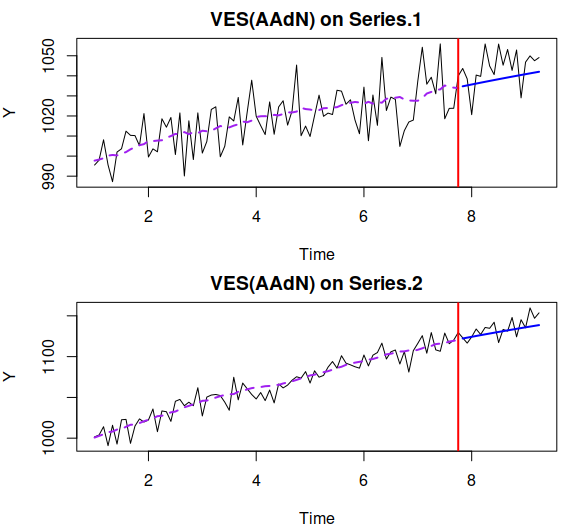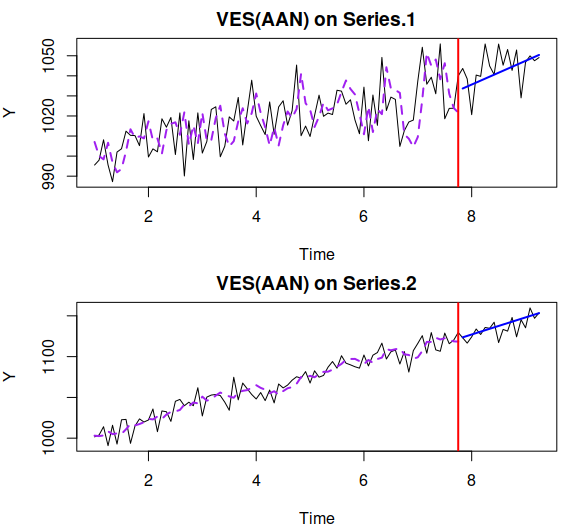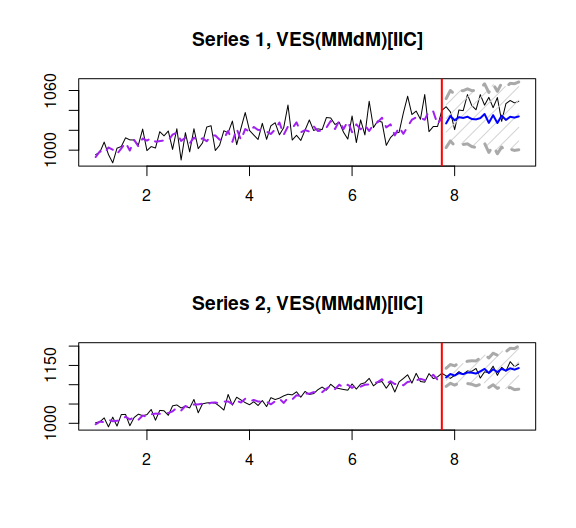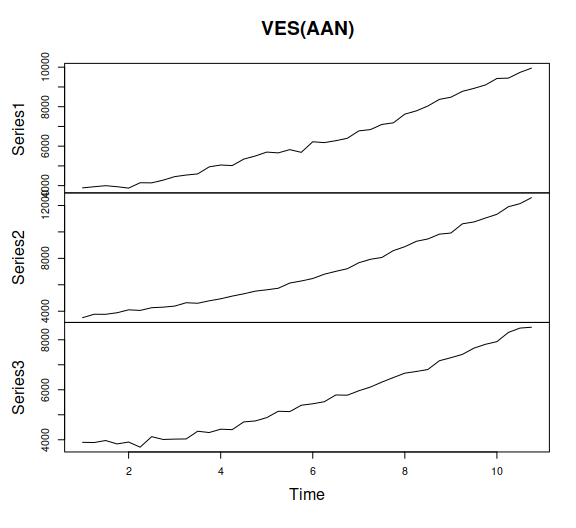# ves() - Vector Exponential Smoothing

#### 2022-02-14

This vignette covers ves() function, which is a part of legion package. In this vignette we will use data from Mcomp package, so it is advised to install it.

require(legion)

We will use to time series from the M3 united in a vector:

Y <- ts(cbind(1000+0.5*c(1:100)+rnorm(100,0,10),
cbind(1000+1.5*c(1:100)+rnorm(100,0,10))),
frequency=12);

ves() function allows constructing Vector Exponential Smoothing (aka “VISTS” discussed by Silva, Hyndman, and Snyder 2010) in either pure additive or pure multiplicative form. The function has several elements that can either be individual or grouped. The former means that all the time series use the same value. For example, persistence="g" means that the smoothing parameters for all the series are the same. A simple call for ves() results in estimation of VES(A,N,N) with grouped smoothing parameters, transition matrix and individual initials:

ves(Y, h=18, holdout=TRUE, silent=FALSE)
## Estimating models... 8 %17 %25 %33 %42 %50 %58 %67 %75 %83 %92 %100 %
## Time elapsed: 2.4047 seconds
##
## Loss function type: likelihood; Loss function value: 615.5072
## Sample size: 82
## Number of estimated parameters: 10
## Number of series: 2
## Number of degrees of freedom per series: 77
## Information criteria:
##      AIC     AICc      BIC     BICc
## 1251.014 1252.736 1275.082 1278.876The output tells us how much time the estimation took, what model we estimated, how many parameters were estimated, the cost function type used and its value and finally the information criteria. Currently we do not provide error measures for the holdout, this functionality will be available with newer releases of legion.

In some cases we may decide that the series should be connected with each other. In this case we can ask function to use “dependent” persistence. This means that along with the individual smoothing parameters, we will estimate cross-series ones. Here’s the example:

ourModel <- ves(Y, "AAN", persistence="d", h=18, holdout=TRUE, silent=FALSE)The resulting persistence matrix contains more values than the individual one:

ourModel$persistence ## Series.1 Series.2 ## Series.1_level 0.53283160 0.11611757 ## Series.1_trend 0.08953404 0.15701303 ## Series.2_level 0.10839086 0.13411503 ## Series.2_trend 0.06591149 0.09006823 Note that some of the values of smoothing parameters are negative and the others are greater than one. This is a normal behaviour for VES model in this implementation. Currently we only have bounds derived from the stability region (bounds="admissible") and we do not do traditional restrictions yet (and not sure if we ever will). Currently we have pure additive and pure multiplicative models only, and I don’t intend introducing mixed models for VES at all, because I think that they are evil. The multiplicative model implemented in VES is in fact just an additive model applied to the data in logarithms. Let’s see how the damped trend multiplicative seasonal model with individual damping and smoothing parameters looks like: ourModel <- ves(Y, "MMdM", phi="i", persistence="i", h=18, holdout=TRUE) We can also produce forecasts from this model: ourForecast <- forecast(ourModel, h=18, interval="prediction") which can then be plotted (if needed): oldpar <- par(mfcol=c(2,1)) plot(ourForecast) par(oldpar)Number of estimated parameters in the model can be extracted via nparam() method. However, when it comes to the calculation of the number of degrees of freedom in the model, this value is divided by the number of series (Lütkepohl 2005). So both ourModel$Sigma and all the information criteria rely on the $$df = T - k_m$$, where $$T$$ is the number of observations and $$k_m = \frac{k}{m}$$ is the number of parameters $$k$$ per series ($$m$$ is the number of series).

AICc and BICc for the vector models are calculated as proposed in (Bedrick and Tsai 1994) and (Tremblay and Wallach 2004).

Currently we don’t do model selection, don’t have exogenous variables and don’t produce conditional prediction interval. But at least it works and allows you to play around with it :).

## Simulate data from VES

There is a function sim.ves(), which allows generating data from VES model:

x <- sim.ves("ANN",frequency=4,obs=40,nvariate=3,randomizer="rnorm",mean=0,sd=100)

This way the we generate two independent time series with similar DGPs and the same parameters (initials, persistence etc). We can use options similar to the ones used in sim.es() from smooth in order to specify a more flexible model.

In addition, we can simulate multivariate series from an estimated VES model:

ourModel <- ves(x\$data,model="AAN",persistence="dependent")
Y <- simulate(ourModel)
plot(Y)When using simulate with ves, you can specify randomizer and additional parameters for distributions. For example, you can use mvrnorm() from MASS package and if you don’t provide mu and Sigma then they will be extracted from the model.